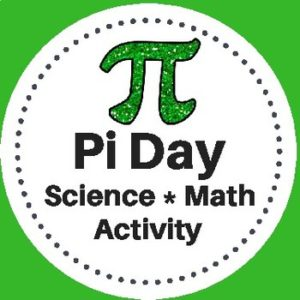Showing all 3 results

•\$1.50

Students will complete a crossword puzzle by answering simple interest word problems. Also included, an optional number search puzzle which will provide an answer bank to use when completing the crossword puzzle! Answer Keys provided.

Sample problem: If you put \$5.62 in a saving account that pays 3% for eight years, what is the amount of money you will have at the end of the eight years?

•\$3.00

This Pi Day Activity has been designed for Science and Math classrooms. Students will use measuring tools to determine the radius, diameter, and circumference of various circular objects. They will then use the data that they collect to calculate pi.

*This activity gives students the opportunity to clearly understand the concept of the number pi and practice math skills through a hands-on activity with a variety of circular objects.

•\$5.00

Here are 250 word problems with answer keys. There are 25 sets, each with 10 problems each.